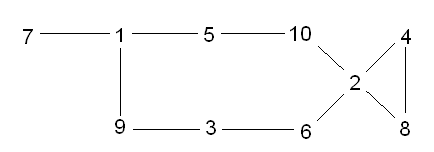#### You may also like### Consecutive Numbers

An investigation involving adding and subtracting sets of consecutive numbers. Lots to find out, lots to explore.### Calendar Capers

Choose any three by three square of dates on a calendar page...### Days and Dates

Investigate how you can work out what day of the week your birthday will be on next year, and the year after...

##### Age 11 to 14 ShortChallenge Level

Build up a long list
$6$
$2$ $6$ $3$
$4$ $2$ $6$ $3$ $9$
$8$ $4$ $2$ $6$ $3$ $9$ $1$
$8$ $4$ $2$ $6$ $3$ $9$ $1$ $5$ $10$
All numbers except $7$ used

Can we also use $7$?
$7$ should go at the end because it can only go next to $1$
$7$ $1$ $?$
$5$ will only fit if $5$ comes next (using the $1$) or if $5$ comes last, after $10$, which would come after $2$

$5$ next:
$7$ $1$ $5$ $10$ $2$ $?$
If $8$ or $4$ used next, $3$, $6$, $9$ won't fit
If $6$ used next, $8$ and $4$ won't fit

$5$ last:
$7$ $1$ $...$ $?$ $2$ $10$ $5$
Exactly the same problem as before now happens before the $2$

We can't use the $7$. We can only use 9 numbers.

The diagram below shows all of the possible connections that can be used.There are many other sequences of nine numbers that follow the rule. Can you find them all?

This problem is taken from the UKMT Mathematical Challenges.
You can find more short problems, arranged by curriculum topic, in our short problems collection.